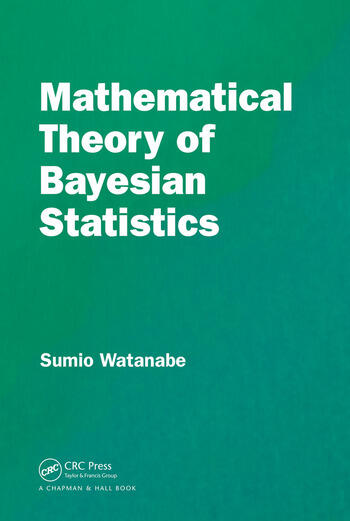# Mathematical Theory of Bayesian Statistics

## 1st Edition

Sumio Watanabe

Chapman and Hall/CRC
Published April 23, 2018
Reference - 320 Pages - 50 B/W Illustrations
ISBN 9781482238068 - CAT# K23205

For Instructors Request Inspection Copy

USD\$169.95

FREE Standard Shipping!

## Preview

### Summary

Mathematical Theory of Bayesian Statistics introduces the mathematical foundation of Bayesian inference which is well-known to be more accurate in many real-world problems than the maximum likelihood method. Recent research has uncovered several mathematical laws in Bayesian statistics, by which both the generalization loss and the marginal likelihood are estimated even if the posterior distribution cannot be approximated by any normal distribution.

Features

• Explains Bayesian inference not subjectively but objectively.
• Provides a mathematical framework for conventional Bayesian theorems.
• Introduces and proves new theorems.
• Cross validation and information criteria of Bayesian statistics are studied from the mathematical point of view.
• Illustrates applications to several statistical problems, for example, model selection, hyperparameter optimization, and hypothesis tests.

This book provides basic introductions for students, researchers, and users of Bayesian statistics, as well as applied mathematicians.

Author

Sumio Watanabe is a professor of Department of Mathematical and Computing Science at Tokyo Institute of Technology. He studies the relationship between algebraic geometry and mathematical statistics.

#### Instructors

We provide complimentary e-inspection copies of primary textbooks to instructors considering our books for course adoption.